Courses

# Test: Engineering Mathematics - 2

## 20 Questions MCQ Test GATE ECE (Electronics) 2022 Mock Test Series | Test: Engineering Mathematics - 2

Description
This mock test of Test: Engineering Mathematics - 2 for Electronics and Communication Engineering (ECE) helps you for every Electronics and Communication Engineering (ECE) entrance exam. This contains 20 Multiple Choice Questions for Electronics and Communication Engineering (ECE) Test: Engineering Mathematics - 2 (mcq) to study with solutions a complete question bank. The solved questions answers in this Test: Engineering Mathematics - 2 quiz give you a good mix of easy questions and tough questions. Electronics and Communication Engineering (ECE) students definitely take this Test: Engineering Mathematics - 2 exercise for a better result in the exam. You can find other Test: Engineering Mathematics - 2 extra questions, long questions & short questions for Electronics and Communication Engineering (ECE) on EduRev as well by searching above.
QUESTION: 1

Solution:
QUESTION: 2

Solution:
QUESTION: 3

### For what value of k, the equation  x+y+z=1 2x+ y+ 4z =k 4x+ y+ 10z k =2 Have a solution

Solution:
QUESTION: 4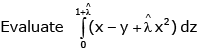along the straight line from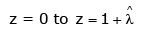Solution:
QUESTION: 5

Consider the differential Equation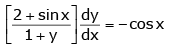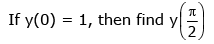Solution:
QUESTION: 6

It is given that  y'' + 2y' + y = 0 y(0) = 0 , y(1) = 0 y(0.5) is _______

Solution:
QUESTION: 7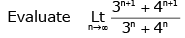Solution:
QUESTION: 8

If the function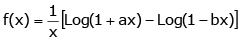is continuous at x = 0 then find f(0)?

Solution:
QUESTION: 9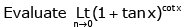Solution:
QUESTION: 10

For what value of k is the following function continuous  x = 0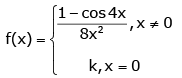Solution:
QUESTION: 11

If y = x |x|, then find Derivative of y?

Solution:
QUESTION: 12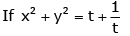and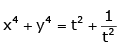find dy/dx

Solution:
QUESTION: 13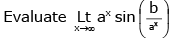Solution:
QUESTION: 14

An insurance company insured 2000 cyclists, 4000 scooter drivers and 6000 motorbike drivers. The probability of an accident involving a cyclist, scooter driver and a motorbike driver are 0.01, 0.03 and 0.15 respectively. One of the insured persons meets with an accident. What is the probability that he is a scooter driver?

Solution:
QUESTION: 15

In answering a question on a MCQ test with 4 choices per question, a student knows the answer, guesses or copies the answer. Let ½ be the probability that he knows the answer, ¼ be the probability that he guesses and ¼ that he copies it. Assuming that a student, who copies the answer, will be correct with the probability ¾, what is the probability that the student knows the answer, given that he answered it correctly?

Solution:
QUESTION: 16

The condition on “a” for which the system of equations

3x − y + 4z = 3, x + 2y − 3z = −2,6x + 5y + az = −3

has a unique solution is

Solution:
QUESTION: 17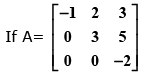then the eigen values of  A3 + 5A + 8I
are

Solution:
QUESTION: 18

The solution of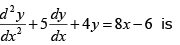Solution:
QUESTION: 19

The differential equation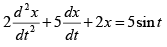given
that x = 2 and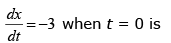Solution:
QUESTION: 20

The next iterative value of the root of x2 − 4 = 0 using the Newton-Raphson method, if the initial guess is 3, is _________

Solution: